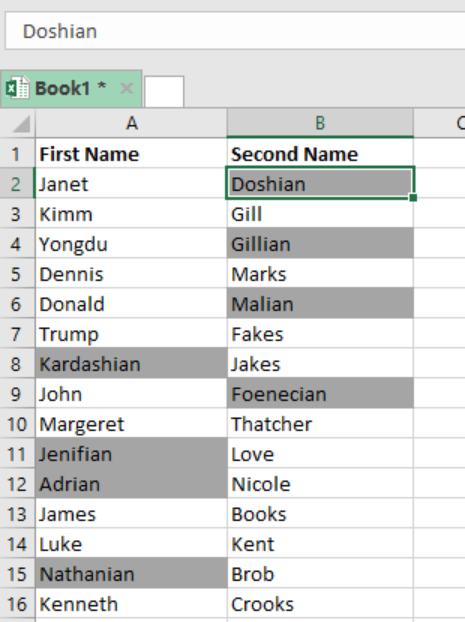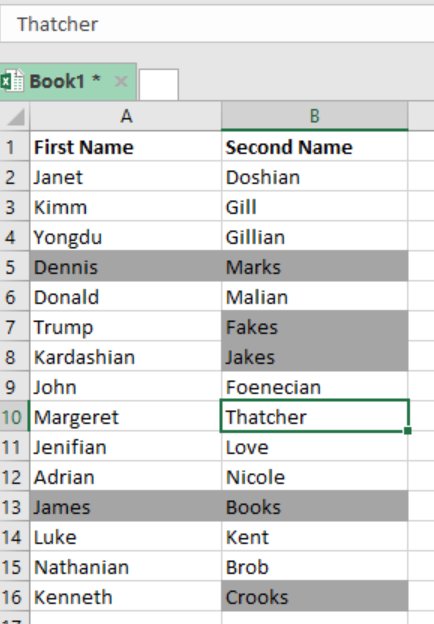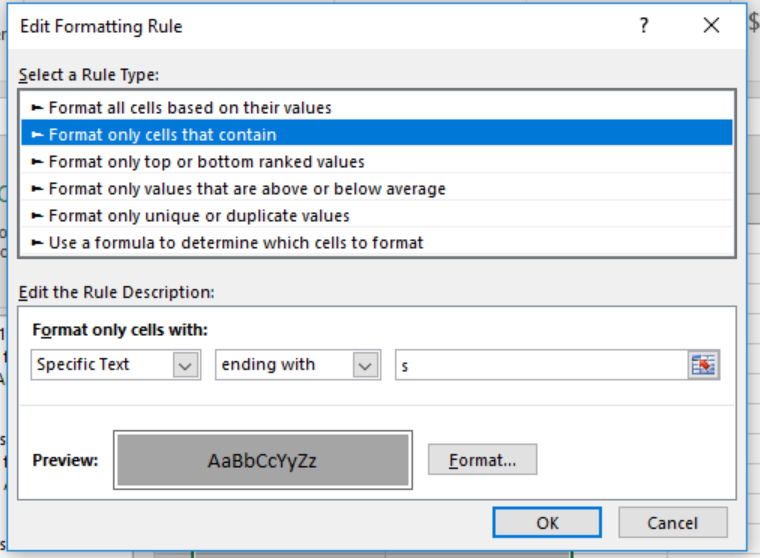Get instant live expert help with Excel or Google Sheets“My Excelchat expert helped me in less than 20 minutes, saving me what would have been 5 hours of work!”

#### Post your problem and you'll get expert help in seconds

Your message must be at least 40 characters
Our professional experts are available now. Your privacy is guaranteed.

# Highlight cells that ends with

We can use a simple formula to highlight cells that end with a certain text in excel. The simple formula should be based on the COUNTIF function. This article looks at how we can highlight cells that begin with a particular text using the COUNTIF function.Figure 1: How to highlight cells that end with a certain text

## General syntax of the formula

`=COUNTIF (A1, “*text”)`

## Understanding the formula

• To highlight cells that end with a particular text, we can apply conditional formatting together with a formula based on the COUNTIF function.
• Note that when applying conditional formatting together with a given formula, the formula is entered relative to the active cell.
• In our case in the example above, our selection is A2:B16, and the active cell among the selection is A2.

## How the formula works

In our case above, the COUNTIF formula will count the number of cells that end with “ian” using a pattern “*ian”. The wildcart (*) is used to match any sequence of characters followed by “ian”.

With conditional formatting, we want a 1 or a zero. Since we are counting one cell at a time, we are sure of getting a 1 or zero for each cell within the range.

• Where we have 1, the rule is triggered and the formatting is applied. Where the result is 0, the rule is not triggered thus nothing happens to the cell.

## How to make the formula more flexible

We can also make the formula more flexible by simply naming an input cell as a named range and then referring to it in the formula. The formula will thus look as below;

`=COUNTIF(B4, “*”&input)`

What this formula does is to add “*” to the beginning of what you put in the input cell. Whenever the value is changed, the conditional formatting will respond.

## ExampleFigure 2: Example of how to highlight cells that end with “s”

In this example, we want to highlight those cells that end with “s”.

To do this, we proceed as follows;

Step 1: Prepare your data in the excel sheet

Step 2: Format the cells with your preferred colorFigure 3: How to apply conditional formatting

Step 3: Press “Ok”

Step 4: Press “Apply” and “Ok” to apply the conditional formatting.

## Instant Connection to an Expert through our Excelchat Service

Most of the time, the problem you will need to solve will be more complex than a simple application of a formula or function. If you want to save hours of research and frustration, try our live Excelchat service! Our Excel Experts are available 24/7 to answer any Excel question you may have. We guarantee a connection within 30 seconds and a customized solution within 20 minutes.

### Did this post not answer your question? Get a solution from connecting with the expert.Another blog reader asked this question today on Excelchat:## Subscribe to Excelchat.coAnother blog reader asked this question today on Excelchat: